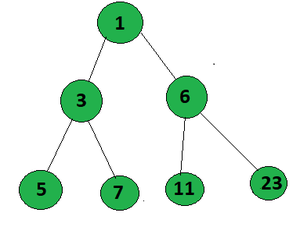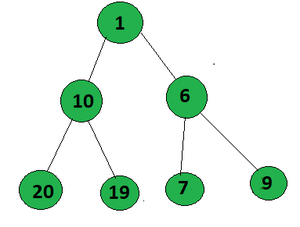# Count of root to leaf paths in a Binary Tree that form an AP

Given a Binary Tree, the task is to count all paths from root to leaf which forms an Arithmetic Progression.

Examples:

Input:Output:
Explanation:
The paths that form an AP in the given tree from root to leaf are:

• 1->3->5 (A.P. with common difference 2)

• 1->6->11 (A.P. with common difference 5)

Input:Output:
Explanation:
The path that form an AP in the given tree from root to leaf is 1->10->19 (A.P. with difference 9)

Approach: The problem can be solved using the Preorder Traversal. Follow the steps below to solve the problem:

• Perform Preorder Traversal on the given binary tree.
• Initialize an array arr[] to store the path.
• Initialize count = 0, to store the count of paths which forms an A.P.
• After reaching the leaf node, check if the current elements in the array(i.e. the node values from root to leaf path) forms an A.P..
• If so, increment the count
• After the complete traversal of the tree, print the count.

Below is the implementation of above approach:

## C++

 `// C++ implementation to count  ` `// the path which forms an A.P.  ` `#include   ` `using` `namespace` `std;  ` ` `  `int` `count = 0;  ` ` `  `// Node structure  ` `struct` `Node {  ` `    ``int` `val;  ` `    ``// left and right child of the node  ` `    ``Node *left, *right;  ` `    ``// intilizaiton consutructor  ` `    ``Node(``int` `x)  ` `    ``{  ` `        ``val = x;  ` `        ``left = NULL;  ` `        ``right = NULL;  ` `    ``}  ` `};  ` ` `  `// Function to check if path  ` `// forma A.P. or not  ` `bool` `check(vector<``int``> arr)  ` `{  ` ` `  `    ``if` `(arr.size() == 1)  ` `        ``return` `true``;  ` ` `  `    ``// if size of arr is greater than 2  ` `    ``int` `d = arr - arr;  ` ` `  `    ``for` `(``int` `i = 2; i < arr.size(); i++) {  ` `        ``if` `(arr[i] - arr[i - 1] != d)  ` `            ``return` `false``;  ` `    ``}  ` ` `  `    ``return` `true``;  ` `}  ` ` `  `// Function to find the maxmimum  ` `// setbits sum from root to leaf  ` `int` `countAP(Node* root, vector<``int``> arr)  ` `{  ` `    ``if` `(!root)  ` `        ``return` `0;  ` ` `  `    ``arr.push_back(root->val);  ` ` `  `    ``// If the node is a leaf node  ` `    ``if` `(root->left == NULL  ` `        ``&& root->right == NULL) {  ` `        ``if` `(check(arr))  ` `            ``return` `1;  ` `        ``return` `0;  ` `    ``}  ` ` `  `    ``// Traverse left subtree  ` `    ``int` `x = countAP(root->left, arr);  ` ` `  `    ``// Traverse the right subtree  ` `    ``int` `y = countAP(root->right, arr);  ` ` `  `    ``return` `x + y;  ` `}  ` ` `  `// Driver Code  ` `int` `main()  ` `{  ` `    ``Node* root = ``new` `Node(1);  ` `    ``root->left = ``new` `Node(3);  ` `    ``root->right = ``new` `Node(6);  ` `    ``root->left->left = ``new` `Node(5);  ` `    ``root->left->right = ``new` `Node(7);  ` `    ``root->right->left = ``new` `Node(11);  ` `    ``root->right->right = ``new` `Node(23);  ` ` `  `    ``cout << countAP(root, {});  ` ` `  `    ``return` `0;  ` `}  `

## Python3

 `# Python3 implementation to count  ` `# the path which forms an A.P. ` ` `  `# Node structure ` `class` `Node: ` `    ``def` `__init__(``self``, x): ` `         `  `        ``self``.val ``=` `x ` `        ``self``.left ``=` `None` `        ``self``.right ``=` `None` `         `  `# Function to check if path  ` `# form a A.P. or not  ` `def` `check(arr): ` `     `  `    ``if` `len``(arr) ``=``=` `1``: ` `        ``return` `True` `     `  `    ``# If size of arr is greater than 2  ` `    ``d ``=` `arr[``1``] ``-` `arr[``0``] ` `     `  `    ``for` `i ``in` `range``(``2``, ``len``(arr)): ` `        ``if` `arr[i] ``-` `arr[i ``-` `1``] !``=` `d: ` `            ``return` `False` `             `  `    ``return` `True` ` `  `# Function to find the maxmimum  ` `# setbits sum from root to leaf  ` `def` `countAP(root, arr): ` `     `  `    ``if` `not` `root: ` `        ``return` `0` `     `  `    ``arr.append(root.val) ` `     `  `    ``# If the node is a leaf node ` `    ``if` `(root.left ``=``=` `None` `and`  `        ``root.right ``=``=` `None``): ` `        ``if` `check(arr): ` `            ``return` `1` `        ``return` `0` `     `  `    ``# Traverse the left subtree ` `    ``x ``=` `countAP(root.left, arr) ` `     `  `    ``# Traverse the right subtree ` `    ``y ``=` `countAP(root.right, arr) ` `     `  `    ``return` `x ``+` `y ` ` `  `# Driver code ` `root ``=` `Node(``1``) ` `root.left ``=` `Node(``3``) ` `root.right ``=` `Node(``6``) ` `root.left.left ``=` `Node(``5``) ` `root.left.right ``=` `Node(``7``) ` `root.right.left ``=` `Node(``11``) ` `root.right.right ``=` `Node(``23``) ` ` `  `print``(countAP(root, [])) ` ` `  `# This code is contributed by stutipathak31jan `

Output:

`2`

Time Complexity: O(N)
Auxiliary Space: O(h), where h is the height of binary tree.

Attention reader! Don’t stop learning now. Get hold of all the important DSA concepts with the DSA Self Paced Course at a student-friendly price and become industry ready.

My Personal Notes arrow_drop_upCheck out this Author's contributed articles.

If you like GeeksforGeeks and would like to contribute, you can also write an article using contribute.geeksforgeeks.org or mail your article to contribute@geeksforgeeks.org. See your article appearing on the GeeksforGeeks main page and help other Geeks.

Please Improve this article if you find anything incorrect by clicking on the "Improve Article" button below.

Improved By : stutipathak31jan